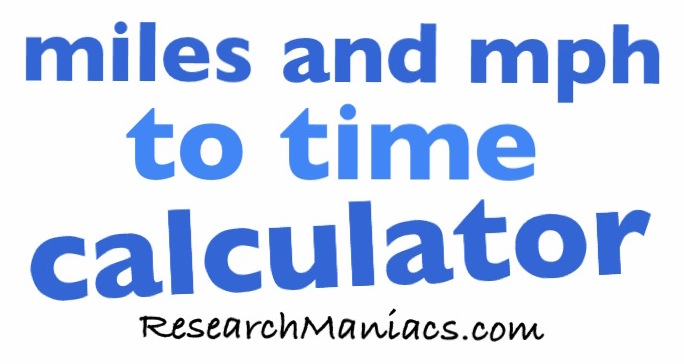How long does it take to drive 370 miles at 60 mph?

 The time it takes to drive 370 miles at 60 miles per hour (mph) is displayed below in hours, minutes, and seconds: 6 hours 10 minutes 0 seconds Do you want to know how to calculate how long it takes to drive 370 miles at 60 mph? No problem. Here is how we did it: 1) Find the number of hours by dividing the distance by mph. The number of hours will be to the left of the decimal point: 370 miles / 60 mph = 6.166666667 = 6 hours 2) Find the number of minutes by multiplying what is remaining from step 1 by 60 minutes. The minutes will be to the left of the decimal point: 0.166666667 x 60 = 10.00000002 = 10 minutes 3) Find the number of seconds by multiplying what is remaining from step 2 by 60 seconds. The seconds will be to the left of the decimal point: 0 x 60 = 0 = 0 seconds How long does it take to drive 370 miles at 61 mph? Here is the next number of miles and mph we converted to time.Miles and mphto Time Calculator Enter another distance (miles) and how fast you are going (mph) below and press "Time to Drive":   miles   mph